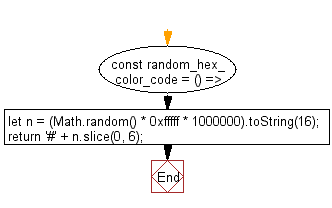# JavaScript: Generate a random hexadecimal color code

## JavaScript fundamental (ES6 Syntax): Exercise-11 with Solution

Write a JavaScript program to generate a random hexadecimal color code.

• Use Math.random() to generate a random 24-bit (6 * 4bits) hexadecimal number.
• Use bit shifting and then convert it to an hexadecimal string using Number.prototype.toString(16).

Sample Solution:

JavaScript Code:

``````//#Source https://bit.ly/2neWfJ2
const random_hex_color_code = () => {
let n = (Math.random() * 0xfffff * 1000000).toString(16);
return '#' + n.slice(0, 6);
};

console.log(random_hex_color_code())
```
```

Sample Output:

```#2375d4
```

Flowchart:Live Demo:

See the Pen javascript-basic-exercise-1-11 by w3resource (@w3resource) on CodePen.

Improve this sample solution and post your code through Disqus

What is the difficulty level of this exercise?

Test your Programming skills with w3resource's quiz.

﻿